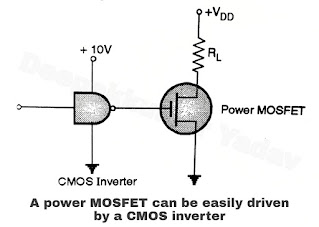Merits, Demerits and Applications of Power MOSFET

Merits of a Power MOSFET (Advantages of MOSFET)

1. The input resistance of MOSFEs is high and they are voltage controlled devices. Therefore the gate driving circuit for MOSFET is simpler.
2. Power MOSFETS can operate at high switching frequency (typically upto 100 kHz). This is due to the fact that they are majority carrier devices.
3. The second. breakdown does not take place in power MOSFETs.
4. MOSFETs have a positive temperature coefficient of resistivity. Therefore. they are the easiest devices to parallel.
5. Due to positive temperature coefficient the MOSFETs to have a better thermal stability (no thermal runaway).
6. It is easy to turn on and off the power MOSFETs.
7. Due to very, high input resistance, the power MOSFET requires extremely small input power and hence it can be driven from CMOS logic gate as shown in Figure.
8. The on state resistance RDS(ON) is extremely small.
9. Power MOSFETs has very low noise figures. So they are less noisy devices as compared to power BJT.
10. The threshold voltage VGS(OFF) ranges from 0.8 to 2 V. Hence MOSFET devices are compatible with TTL logic.
11. Due to the drift layer the breakdown voltages of power MOSFETs are high as compared to small signal MOSFET.Demerits of a Power MOSFET (Disadvantages of MOSFET)
1. The on state voltage across a power MOSFET is high. Therefore the on state power dissipation is very high, as compared to that of a power transistor.
2. Power MOSFETS need special care while handling otherwise they can get damaged due to static electricity.
3. Power MOSFETS have asymmetric blocking capacity. They can block a high forward voltage but they cannot block high reverse voltage. Therefore to protect the power MOSFET we need to connect a diode across the MOSFET as shown in Figure.Applications of Power MOSFET
1. In high frequency inverter.
2. In the switched mode power supplies (SMPS).
3. In uninterruptable power supplies (UPS).
4. In the output stage of audio or RF power amplifier.
5. Industrial process control.
6. Motor control applications.
7. Solenoid or relay driver.
8. Display driver.